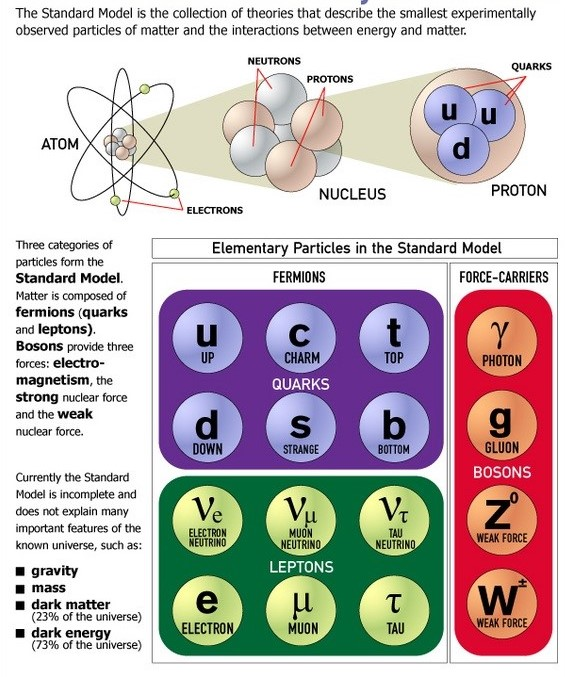# What are W Bosons?

Note4Students

From UPSC perspective, the following things are important :

Prelims level : Various sub-atomic particles

Mains level : Particle physicsResearchers from Collider Detector at Fermilab (CDF) Collaboration, in the US, announced that they have made a precise measurement of the mass of the so-called W boson.

Do you know?

There are four fundamental forces at work in the universe: the strong force, the weak force, the electromagnetic force, and the gravitational force. They work over different ranges and have different strengths. Gravity is the weakest but it has an infinite range.

#### What is W Boson?

• Discovered in 1983, the W boson is a fundamental particle.
• Together with the Z boson, it is responsible for the weak force, one of four fundamental forces that govern the behaviour of matter in our universe.
• Particles of matter interact by exchanging these bosons, but only over short distances.
• The W boson, which is electrically charged, changes the very make up of particles.
• It switches protons into neutrons, and vice versa, through the weak force, triggering nuclear fusion and letting stars burn.
• This burning also creates heavier elements and, when a star dies, those elements are tossed into space as the building blocks for planets and even people.

#### Debate over W Boson’s mass

• The weak force was combined with the electromagnetic force in theories of a unified electroweak force in the 1960s, in an effort to make the basic physics mathematically consistent.
• But the theory called for the force-carrying particles to be massless, even though scientists knew the theoretical W boson had to be heavy to account for its short range.
• Theorists accounted for the mass of the W by introducing another unseen mechanism. This became known as the Higgs mechanism, which calls for the existence of a Higgs boson.

#### What is the news?

• CDF researchers stated that this precisely determined value did not match with what was expected from estimates using the standard model of particle physics.
• This result is highly significant because this implies the incompleteness of the standard model description.
• This is a major claim, since the standard model has been extraordinarily successful in the past decades.
• Hence, physicists are looking for corroboration from other, independent, future experiments.

#### What is the standard model of elementary particle physics?

• The Standard Model of particle physics is the theory describing three of the four known fundamental forces (the electromagnetic, weak, and strong interactions while omitting gravity) in the universe and classifying all known elementary particles.
• It is a theoretical construct in physics that describes particles of matter and their interaction. Ex. Proton, Neutron, Electron etc.
• It is a description that views the elementary particles of the world as being connected by mathematical symmetries, just as an object and its mirror image are connected by a bilateral (left–right) symmetry.
• These are mathematical groups generated by continuous transformations from, say, one particle to another.
• According to this model there are a finite number of fundamental particles which are represented by the characteristic “eigen” states of these groups.
• The particles predicted by the model, such as the Z boson, have been seen in experiments and the last to be discovered, in 2012, was the Higgs boson which gives mass to the heavy particles.

#### Why is the standard model believed to be incomplete?

• The standard model is thought to be incomplete because it gives a unified picture of only three of the four fundamental forces of nature and it totally omits gravity.
• So, in the grand plan of unifying all forces so that a single equation would describe all the interactions of matter, the standard model was found to be lacking.
• The other gap in the standard model is that it does not include a description of dark matter particles.

#### How are the symmetries related to particles?

• The symmetries of the standard model are known as gauge symmetries, as they are generated by “gauge transformations” which are a set of continuous transformations (like rotation is a continuous transformation).
• Each symmetry is associated with a gauge boson.
• For example, the gauge boson associated with electromagnetic interactions is the photon.
• The gauge bosons associated with weak interactions are the W and Z bosons. There are two W bosons — W+ and W-.

#### What is the main result of the recent experiment?

• The recent experiment at CDF, which measured the mass of the W boson as 80,433.5 +/- 9.4 Mev/c2, which is approximately 80 times the mass of a hydrogen nucleus.
• This came out to be more than what is expected from the standard model.
• The expected value using the standard model is 80,357 +/- 8 MeV/c2 .
• Thus, the W boson mass itself is a prediction of the standard model.
• Therefore, any discrepancy in its mass means a lack of self-consistency in the standard model.

#### What is the discrepancy they obtained?

• The mass discrepancy of the W boson needs to be checked and confirmed to the same accuracy by other facilities, for example, the Large Hadron Collider (LHC).

#### Where do we stand now in terms of new physics?

• New physics is in the air, and experiments have been gearing up for some years now to detect new particles.
• With its high-precision determination of the W boson mass, the CDF has struck at the heart of the standard model.
• So it is a significant finding and if this is confirmed by the LHC and other experiments, it will throw open the field for ideas and experiment.